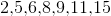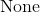Example Questions

← Previous 1

Example Question #1 : Mode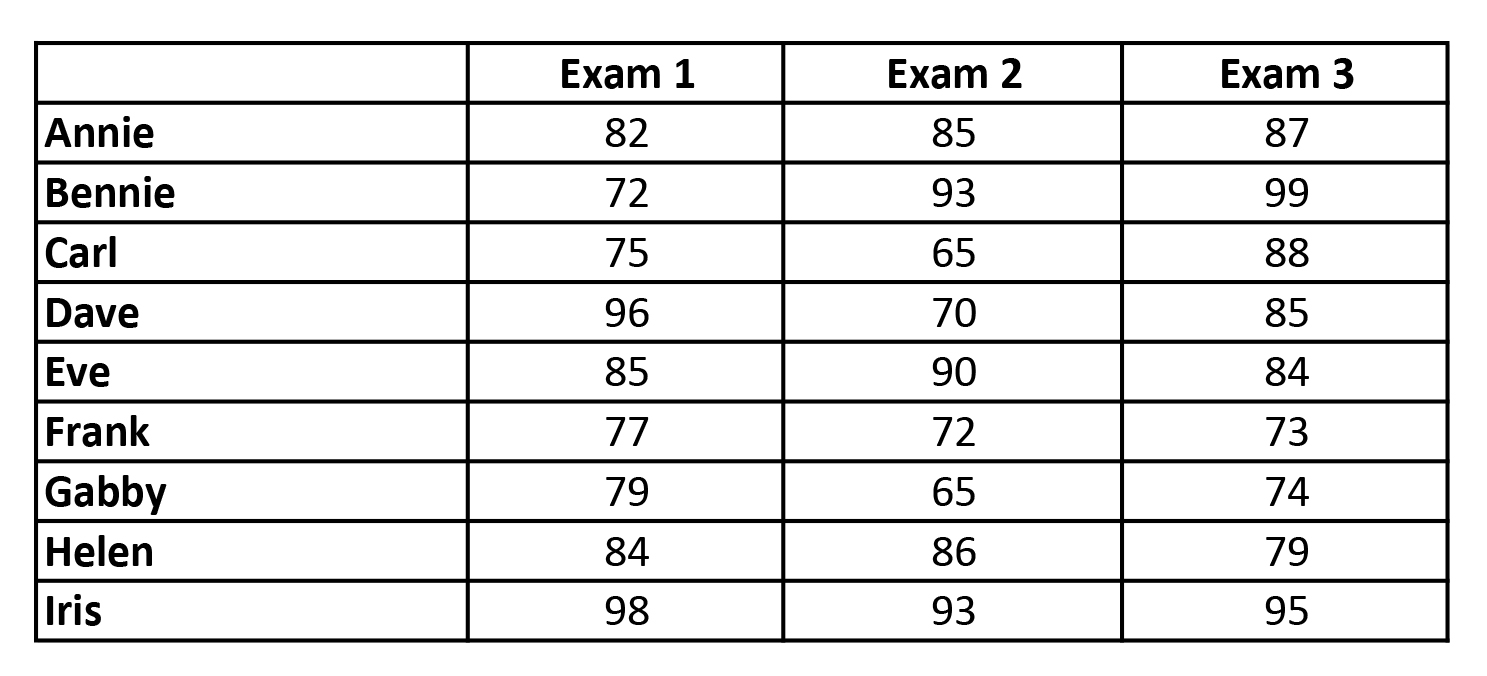Refer to the chart above.  What is the mode for all of the exam scores?

84

83

72

77

85

85

Explanation:

Mode is the value that occurs most often within a set of data.

Example Question #1 : How To Find Mode

What is the sum of the mean, mode, and median of the following set of data:

20, 25, 7, 40, 13, 15, 20, 28

52

40

61

20

75

61

Explanation:

First, the data set must be put in order from smallest to largest

7, 13, 15, 20, 20, 25, 28, 40

Mean = sum of data ÷ number of data points = 21

Mode = number occuring most often = 20

Median = middle number, or the mean of the two middle numbers = 20

Therefore, the sum of the mean, mode, and median is 61

Example Question #3 : How To Find Mode

What is the mode of the following set of data?

{1, 3, 5, 3, 1, 6, 7, 3}

2

6

3

5

1

3

Explanation:

The most common element of the set of data is 3. It occurs three times, and no other number occurs 3 times.

Example Question #4 : How To Find Mode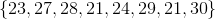What is the mode of this set of numbers?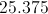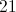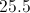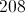Explanation:

The mode is the most commonly occuring number in a group of numbers.is the only number that occurs more than once.

Example Question #1 : How To Find Mode

What is the mode of the following list of test scores: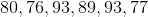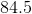There is no mode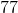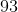Explanation:

The mode is the most frequently occurring number. Thus it is 93 as that is the only repeated number.

Example Question #6 : How To Find Mode

The following is a list of the ages ofpeople randomly selected off the street: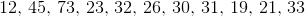What is the mode of the data set?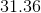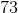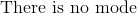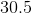Explanation:

The mode is the most frequently occuring number in the data set. This data set has only unique numbers, so there is no frequently occuring number. Thus there is no mode.

Example Question #7 : How To Find Mode

A student has been taking practice ACT tests all summer. He has taken a total of eight tests and recorded the following composite scores: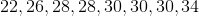.

What was the mode value of his scores?Explanation:

The mode is the data point that occurs the most frequently in a group of data.

If you look at our 8 data points, only two values occur more than once. Those values are 28 and 30. 28 occurs twice and 30 occurs three times.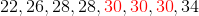Therefore, 30 is the mode.

Example Question #8 : How To Find Mode

The following is a list of days of the month that the new moon was in the sky: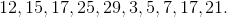What is the mode?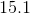Explanation:

The mode is the most frequently occurring number in a data set. Sinceis the only repeated number, it is the mode.

Example Question #9 : How To Find Mode

What is the mode of the following list of numbers: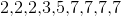?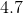Explanation:

The mode is the number that occurs the most. Sinceoccurs more thanand any other number, it is the mode.

Example Question #10 : How To Find Mode

Find the mode of the following set of numbers.